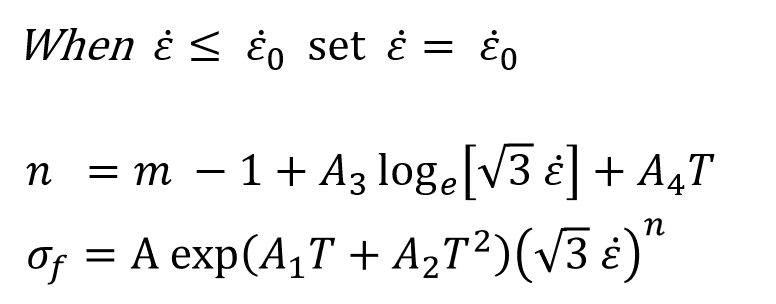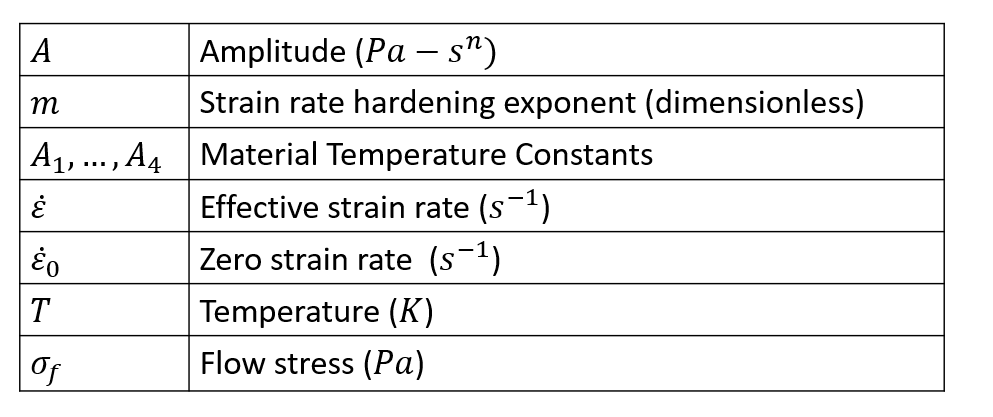# Norton-Hoff Model

Temperature, strain, and strain rate dependent Norton-Hoff model used in HyperXtrude is given by the following expression.Workpiece Aluminum { ConstitutiveModel = "PowerLaw" Density = ρ SpecificHeat = Cp (T) Conductivity = K (T) CoeffOfThermalExpansion = βT VolumetricHeatSource = Qvol Amplitude = μ0 ConstantY = Y StressRateHardeningExponent = m ReferenceTemperature = T0 SolidusTemeprature = Ts LiquidusTemeprature = T1 TemperatureDependence = "Exp (A1T+A2T^2)" StrainOffset = ε TemperatureConstantA1 = A1 TemperatureConstantA2 = A2 TemepratureConstantA3 = A3 TemperatureConstnatA4 = A4 YoungModulus = E PoissonRatio = ν }